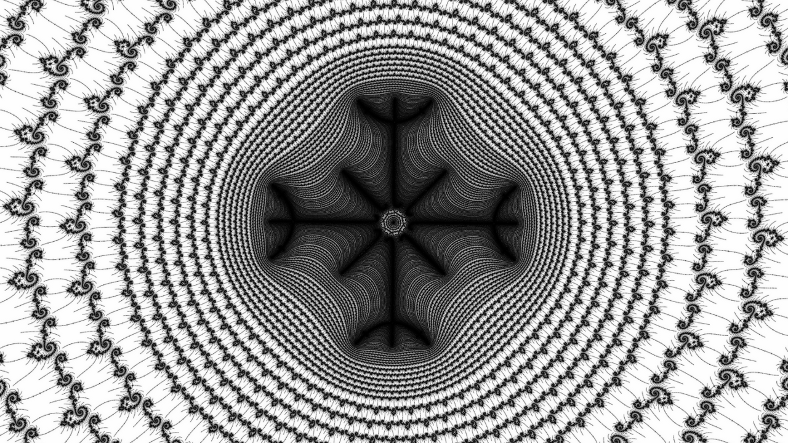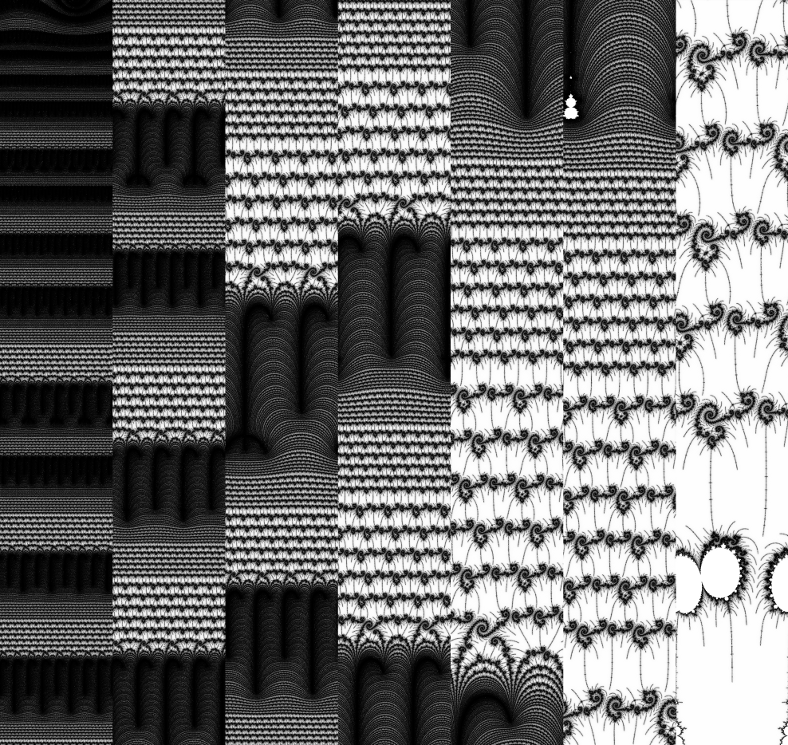# mathr / blog / #

## Exponential mapping with Kalles FraktalerAbove, a standard flat view of part of the Mandelbrot set. Below, a zoom through that location unwrapped into log-polar coordinates.The program I wrote to transform a raw zoom out sequence from Kalles Fraktaler 2 into an exponential map PGM is available, along with some other utilities and a mini-library that might make it easier for you to write your own:

git clone http://code.mathr.co.uk/kf-extras.git
browse https://gitorious.org/maximus/kf-extras http://code.mathr.co.uk/kf-extras

How it works isn't too complex (most of the effort I put into making it stream data so it doesn't run out of memory for huge zoom sequences). The key loop that converts the flat image into a log-polar image runs like this:

  #pragma omp parallel for
for (int k = 0; k < expmap->circumference; ++k) {
double r = pow(0.5, 1.0 - expmap->row / (double) expmap->radius);
double t = 2 * pi * k / (double) expmap->circumference;
double i = r * cos(t) * expmap->height / 2.0 + expmap->width / 2.0;
double j = r * sin(t) * expmap->height / 2.0 + expmap->height / 2.0;
row[k] = kfb_get_nearest(expmap->kfb, i, j);
}
expmap->row++;

Here width and height refer to the flat image, while circumference is the width of the output strip, and radius is the height of each section (one section is generated for each input file). The expmap->row variable is a counter that loops through concentric circles in the flat image, and there's some relatively simple maths to calculate the radius and angle for each point on the circle. Calculating the circumference and radius parameters is a bit more involved, here's the bit of code that does that with a few inline comments:

    expmap->circumference = expmap->height * pi / 2;
// 0.5 ^ (1 - 1 / radius) - 0.5 = |(0.5, 0)-(0.5*cos(t), 0.5*sin(t))|
//    where t = 2 * pi / circumference
double t = 2 * pi / expmap->circumference;
double dx = 0.5 - 0.5 * cos(t);
double dy = 0.5 * sin(t);
double d = sqrt(dx * dx + dy * dy);
// (1 - 1 / radius) log 0.5 = log (d + 0.5)
// 1 - 1 / radius = log (d + 0.5) / log 0.5
// 1 / radius = 1 - log (d + 0.5) / log 0.5
expmap->radius = 1.0 / (1.0 - log(d + 0.5) / log(0.5));

The idea behind the maths is that ideally we want a uniform pixel aspect ratio, with pixels as square as possible. There's probably a neater and more direct way of calculating this, but I got it working so stopped looking for a better solution.

Then the main loop steps through one row at a time (loading successive kfb files as necessary), keeping the previous row around to do the pseudo-de colouring I described in the previous blog post.